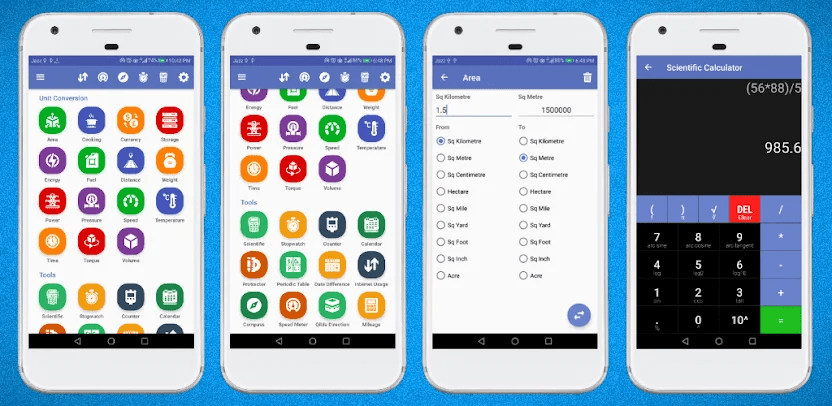# All in One Unit Converter Pro APK (Paid/Full)

3.4.0
• Payment
All in One Unit Converter Pro - Calculator is a comprehensive and powerful tool designed to meet the needs of engineers and professionals
Developer
Techsial Android Extreme Tech Arena
Updated
Jun 17, 2023
Version
3.4.0
Requirements
6.0
1,000+
Report this app

## Description

All in One Unit Converter Pro – Calculator is an advanced engineering unit conversion calculator that supports both Metric and Imperial Units. It offers a wide range of smart tools that are highly useful for engineers and professionals in various fields.## Conversions Tab:

• Length/Distance Unit Converter: Convert between units such as Kilometer to Mile, Foot to Inches, and more.
• Area Units Converter: Convert units like Hectare to Acre, Mile to Yard, and others.
• Volume Unit Converter: Easily convert units like Cubic Meter to Cubic Centimeter and vice versa.
• Mass/Weight Unit Converter: Convert units like Kilogram to Pounds, Grams to Ounces, and more.
• Cooking Unit Converter: Convert units commonly used in cooking, such as Teaspoon to Tablespoon, Cup to Teaspoon, and others.
• Computer Storage Units Converter: Convert units like Bit to Byte, Megabyte to Kilobyte, and more.
• Temperature Units Converter: Convert between Fahrenheit, Celsius, and Kelvin scales.
• Time Unit Converter: Convert units like Year to Month, Seconds to Nanoseconds, and more.
• Fuel Units Converter: Convert units like Kilometers per Litre to Miles per Litre.
• Speed Units Converter: Convert units of speed, such as Mile per Hour to Kilometer per Hour.
• Pressure Unit Converter: Convert units like Kilopascal to Pascal, Torr to Pascal, and more.
• Force Calculator: Use the force formula to convert units like Newton to Dyne.
• Torque Units Converter: Convert units used to measure torque.
• Angle Calculator: Convert between Degree and Radian units.
• Density Calculator: Calculate density using the appropriate formula.
• Viscosity Unit Conversion: Convert units of viscosity.
• Flow and Surface Tension: Convert units related to flow and surface tension.
• Energy Unit Converter: Convert units of energy, such as Joule to Calorie, Kilojoule to Joule, and more.
• Power Units Converter: Convert units of power, for example, Megawatt to Kilowatt.
• Electric Current, Electric Resistance, Electric Conductance, Electrical Resistivity, and Electrical Conductivity: Convert various electrical units.
• Capacitance of Capacitor: Convert capacitance units.
• Inductance of Inductor: Convert inductance units.
• Electric Charge Converter: Convert electric charge units.
• Electric Potential, Sound Unit Converter, Frequency Conversion, and Screen Resolution: Convert units in these respective categories.

## Tools Tab:

• Scientific Calculator: Perform complex scientific calculations.
• Stop Watch: Convenient tool for timing activities.
• Memory Usage: Monitor and assess memory usage.
• Protractor: Measure and calculate angles.
• Periodic Table: Access the periodic table of elements.
• Number Counter: Count numbers and keep track of the total.
• Compass Needle: Use a virtual compass needle.
• Speed Meter: Measure speed accurately.
• RGB to HEX Converter and HEX to RGB Converter: Convert color codes between RGB and HEX formats.
• Qibla Direction Finder: Determine the direction of Qibla.
• Calendar: Access a calendar for date-related calculations.
• Timer: Set timers and manage countdowns.

## Calculations Tab:

• Area Calculator, Surface Area Calculator, Perimeter Calculator: Calculate areas, surface areas, and perimeters.
• Volume Calculator: Calculate volume using the appropriate formula.
• Permutation Calculator: Calculate permutations.
• Decimal to Fraction Calculator: Convert decimals to fractions.
• Proportion Calculator: Solve proportions.
• Date Difference Calculator (Age Calculator): Calculate the difference between dates, often used as an age calculator.
• Mileage Calculator, Distance Calculator, Fuel Calculator: Calculate mileage, distance, and fuel consumption.

The Unit Converter App offers an extensive range of unit conversions and useful tools, making it a valuable resource for engineers and professionals in various disciplines.

## Conclusion:

All in One Unit Converter Pro – Calculator is a comprehensive and powerful tool designed to meet the needs of engineers and professionals who frequently encounter unit conversions and scientific calculations. With support for both Metric and Imperial Units, it offers a wide range of conversion categories, including length, area, volume, mass/weight, cooking units, computer storage, temperature, time, fuel, speed, pressure, force, torque, angle, density, viscosity, flow, surface tension, energy, power, electric units, capacitance, inductance, electric charge, sound, frequency, and screen resolution.

## What's new

- 'Text to Speech' converter is added in 'Tools' section
- 'Micro Newton' unit is added in 'Force' conversions
- 'Electron-Volt' and 'Therm' units are added in Energy conversions

## Package Info:

★ Untouched Paid apk with Original Hash Signature
★ Certificate MD5 digest: cabe82ee8cf53fd803de1ffe7513e798
★ No changes were applied
➡ Languages: Full Multi Languages
➡ CPU architectures: Universal
➡ Screen DPIs: 120dpi, 160dpi, 240dpi, 320dpi, 480dpi, 640dpi